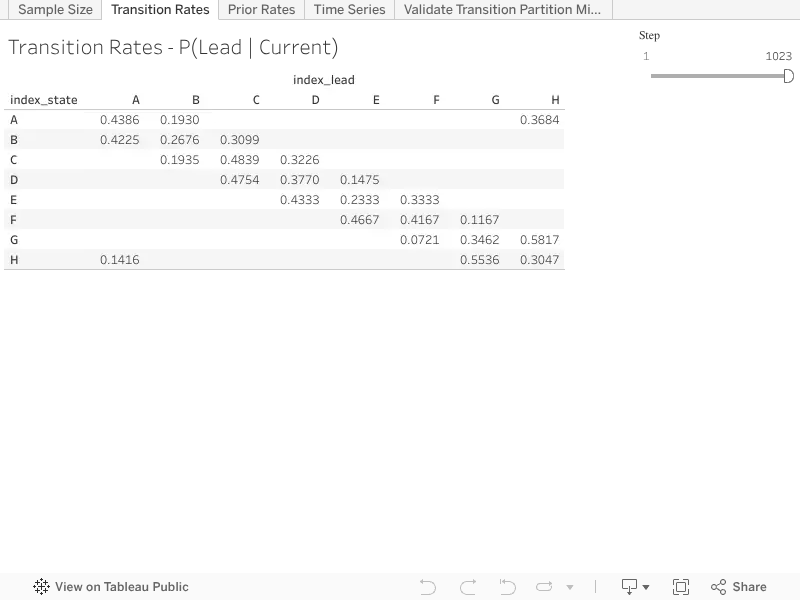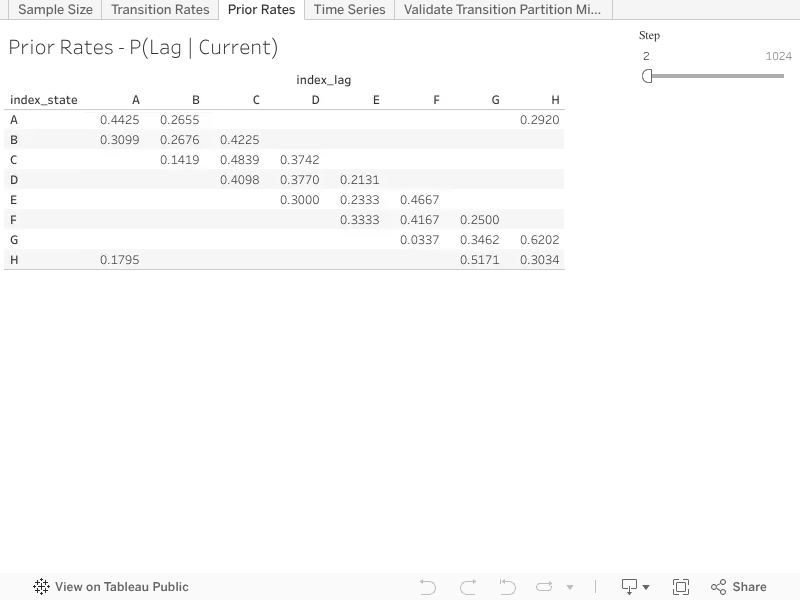# LEAD and LAG using level of detail calculations

Version 9

Description:

A common task in longitudinal analysis is to compare an observed [VALUE] to either a previous [LAG] or following [LEAD] value with respect to a prescribed [ORDER]. With the introduction of level of detail calculations referential calculated fields, that were once only possible on aggregates using the LOOKUP() table calculation, can now be created on granular records, with little more than simple binary congruence modular arithmetic.

To implement the calculation described in this article you have to create a field in the data source, outside of Tableau, that contains a non-negative unit increment ordering (i.e 0, 1, 2, 3, etc...) of every single record. This ordering need not be unique in the whole data source, as long at it is unique within the windowing partition in which the [LEAD] and [LAG] are being calculated. For the sake of clarity we will develop the calculation in this article assuming the [LEAD] and [LAG] will be calculated over the whole data set. In this article [ORDER] will denote ordering field, and [VALUE] will denote the field containing the values for which we seek to calculate the [LEAD] and [LAG].

The heart of the calculation is found in realizing that the [LEAD] level of detail is calculated over the two record sub-partition window starting with the current record, and the [LAG] level of detail is calculated over the two record sub-partition window ending in the current record. Next note that there are two possible sub-partition windows containing two records, those starting on even [ORDER] records found by FLOOR([ORDER] / 2), and those starting on odd order records found by CEILING([ORDER] / 2). Combining these two insights together we can search for the [LEAD] and [LAG] values using the appropriate sub-partition windows. The required sub-partition window for the level of detail calculations is summarized in the following table.

[ORDER] % 2 = 0
FLOOR([ORDER] / 2)CEILING([ORDER] / 2)
[ORDER] % 2 = 1CEILING([ORDER] / 2)FLOOR([ORDER] / 2)

The binary congruence and sub-partition windows of the [LEAD] and [LAG] level of detail calculations are illustrated in the following 10 record example.

[ORDER][VALUE]
[ORDER] % 2FLOOR([ORDER] / 2)
CEILING([ORDER] / 2)
0A000B
1B101CA
2C011DB
3D112EC
4E022FD
5F123GE
6G033HF
7H134IG
8I044JH
9J145I

The final component to the level of detail calculations is to ensure that we only return the [VALUE] of the required record, which can be done by nulling out records of the same congruence as the current record, nulling even [ORDER] records if the [ORDER] of the current record is even, and odd [ORDER] records if the [ORDER] of the current record is odd.

Example Calculation:

To find the following [VALUE] we need to look forward in the binary window in which the current [ORDER] is the lowest, while assigning null to the current [VALUE].

// There is no following observation after the last observation.

[LEAD] := IF [ORDER] >= { FIXED : MAX([ORDER]) } THEN

NULL

// Even ordered observation use the even based windowed to look forward.

// Note that the aggregate is superfluous, because we are nulling out

// the even ordered observation.

ELSEIF [ORDER] % 2 = 0 THEN

{ FIXED FLOOR([ORDER] / 2) : MAX(IIF([ORDER] % 2 = 1, [VALUE], NULL)) }

// Odd ordered observation use the odd based windowed to look forward.

// Note that the aggregate is superfluous, because we are nulling out

// the odd ordered observation.

ELSE

{ FIXED CEILING([ORDER] / 2) : MAX(IIF([ORDER] % 2 = 0, [VALUE], NULL)) }

END

To find the previous [VALUE] we need to look backward in the binary window in which the current [ORDER] is the highest, while assigning null to the current [VALUE].

// There is no previous observation before the first observation.

[LAG] := IF [ORDER] <= { FIXED : MIN([ORDER]) } THEN

NULL

// Even ordered observation use the odd based windowed to look back.

// Note that the aggregate is superfluous, because we are nulling out

// the even ordered observation.

ELSEIF [ORDER] % 2 = 0 THEN

{ FIXED CEILING([ORDER] / 2) : MIN(IIF([ORDER] % 2 = 1, [VALUE], NULL)) }

// Odd ordered observation use the even based windowed to look back.

// Note that the aggregate is superfluous, because we are nulling out

// the odd ordered observation.

ELSE

{ FIXED FLOOR([ORDER] / 2) : MIN(IIF([ORDER] % 2 = 0, [VALUE], NULL)) }

END

Inputs and Setup:

The [ORDER] of the records is a unit incremented non-negative integer field, that must be assigned outside of Tableau when the data set is constructed. The [VALUE]can be any field or calculation that is allowed in an aggregate. The [LEAD] and [LAG] can be further constrained to smaller partitions, for example by customer, facility, or state, by adding the same additional dimensions to the window partition clause of the every level of detail calculation.

The level of detail calculations partition their even and odd based windows over the calculations FLOOR([ORDER] / 2) and CEILING([ORDER] / 2) respectively.

While generalizing this method to N steps is possible, in practice the coding is cumbersome and brittle due to the unwieldy requirement to build N+1 branches into the condition statement.

Related Functions:

CEILING(), FLOOR(), IIF(), %

The [LEAD] calculation is illustrated in the following worksheet of forward transition probabilities.The [LAG] calculation is illustrated in the following worksheet of backward prior probabilities.Finding the [LEAD] and [LAG] is a foundational requirement of auto-regression, particular in the analysis of stochastic processes. The source code to generate the sample data is available in a Jupyter notebook hosted on GitHub.